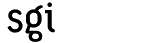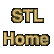# reverseCategory: algorithms Component type: function

### Prototype

```template <class BidirectionalIterator>
void reverse(BidirectionalIterator first, BidirectionalIterator last);
```

### Description

Reverse reverses a range. That is: for every i such that 0 <= i <= (last - first) / 2), it exchanges *(first + i) and *(last - (i + 1)).

### Definition

Defined in the standard header algorithm, and in the nonstandard backward-compatibility header algo.h.

### Preconditions

• [first, last) is a valid range.

### Complexity

Linear: reverse(first, last) makes (last - first) / 2 calls to swap.

### Example

```vector<int> V;
V.push_back(0);
V.push_back(1);
V.push_back(2);
copy(V.begin(), V.end(), ostream_iterator<int>(cout, " "));
// Output: 0 1 2
reverse(V.begin(), V.end());
copy(V.begin(), V.end(), ostream_iterator<int>(cout, " "));
// Output: 2 1 0
```

### Notes